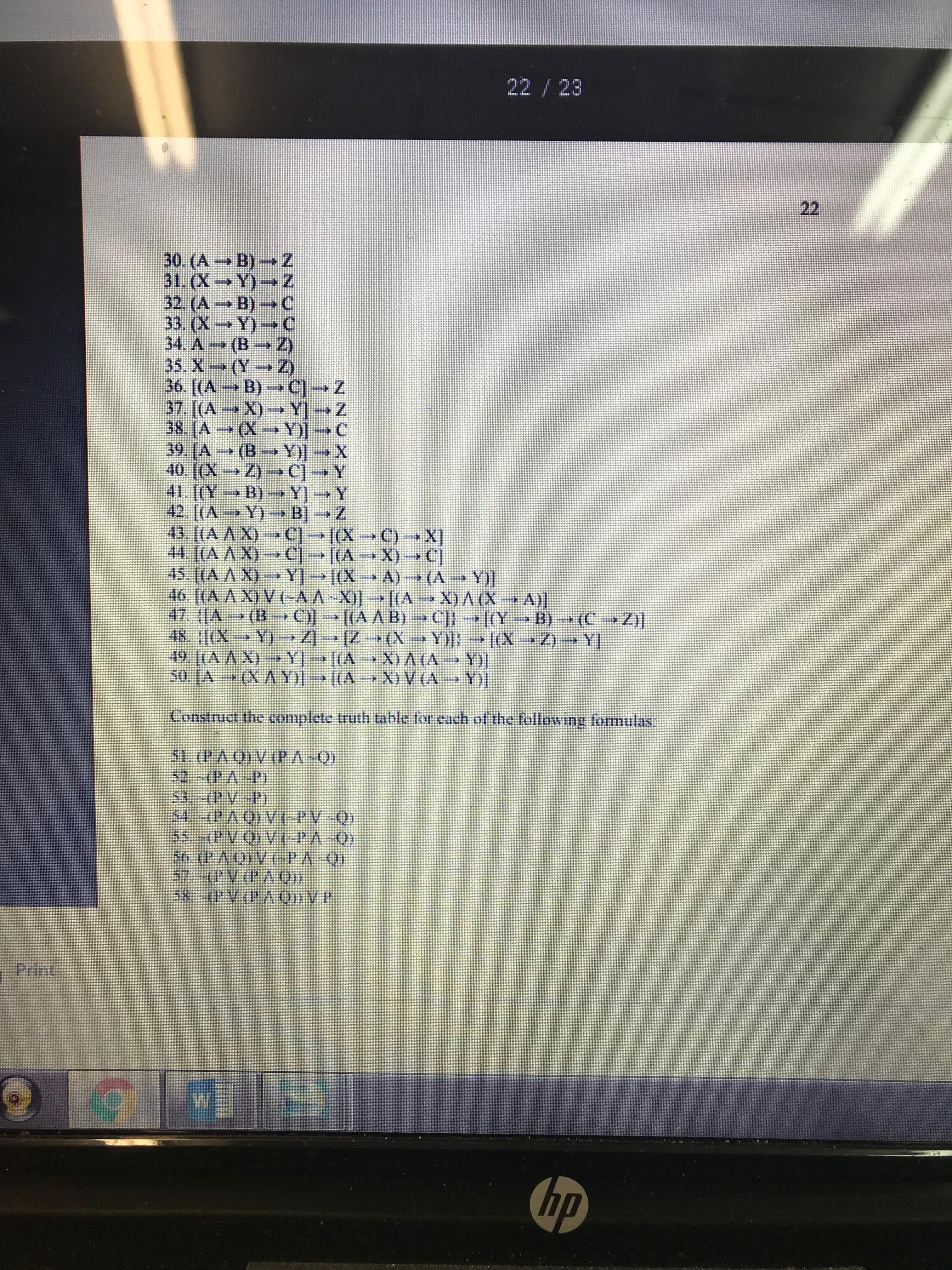# 22 2330 (A B) Z31,(X-Y)-→ Z32. (А-В-С33 (X -→ Y)-→ C34. A → (B-+2)36. (A → B) → C1 → Z38. IA (X-Y)-CConstruct the complete truth table for each of the following formulas51 (P AO) V(PA-O)57 (PV(PAO)Print

Question

Compute the truth values of the following symbolic statements, supposing that the truth value
of A, B, C is T, and the truth value of X, Y, Z is F.
#36help_outlineImage Transcriptionclose22 23 30 (A B) Z 31,(X-Y)-→ Z 32. (А-В-С 33 (X -→ Y)-→ C 34. A → (B-+2) 36. (A → B) → C1 → Z 38. IA (X-Y)-C Construct the complete truth table for each of the following formulas 51 (P AO) V(PA-O) 57 (PV(PAO) Print fullscreen
check_circleExpert Solution
Step 1

36). Since true value of A is T and B is T then A→B will be T because in implication only T → F is false.

Step 2

Similarly (A→B) →C is true.

Step 3

In the same way {(A→B) →C}&ra...

### Want to see the full answer?

See Solution

#### Want to see this answer and more?

Solutions are written by subject experts who are available 24/7. Questions are typically answered within 1 hour*

See Solution
*Response times may vary by subject and question
Tagged in

### Other